﻿ 关于影响人民币国际储备地位主要因素的实证分析 An Empirical Analysis on the Determinants of the International Reserve Status of RMB

Emergence and Transfer of Wealth
Vol. 08  No. 03 ( 2018 ), Article ID: 26719 , 11 pages
10.12677/ETW.2018.83004

An Empirical Analysis on the Determinants of the International Reserve Status of RMB

Chunling Huang, Yuping Lan

International Business Faculty, Beijing Normal University, Zhuhai Guangdong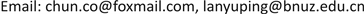Received: Aug. 15th, 2018; accepted: Aug. 30th, 2018; published: Sep. 6th, 2018ABSTRACT

This paper analyzes the current situation of the RMB, respectively from five aspects: economic strength, internal and external currency stability, international trade strength, foreign exchange reserves, and financial market development, and the determining factors that affect the share of the international reserve currency by employing regression analysis of the SPSS software, which use data including eight indexes during the year of 2000 to the year of 2015 RMB. Using time series model to predict the internationalization of the RMB on the year of 2018, 2019 and 2020, We get the empirical conclusion of the determining factors on the development of the status of RMB and put forward related suggestions to this specific situation.

Keywords:RMB, Regression Analysis, Currency International Reserve Status, Exponential Smoothing, The Financial MarketsCopyright © 2018 by authors and Hans Publishers Inc.

This work is licensed under the Creative Commons Attribution International License (CC BY).

http://creativecommons.org/licenses/by/4.0/1. 引言及文献综述

2. 研究方法

3. 模型与数据分析

3.1. 变量说明(表1)

1) 基本经济情况

2) 金融市场发展

3) 币值稳定性

4) 网络外部性

3.2. 模型建立

$R\text{esv}=\alpha +{\beta }_{1}gdp+{\beta }_{2}iex+{\beta }_{3}fdi+{\beta }_{4}kpn+{\beta }_{5}stock+{\beta }_{6}\mathrm{inf}+{\beta }_{7}reer+{\beta }_{8}lagresv+u$

3.3. 实证假设(表2)

3.4. 参数估计(表3)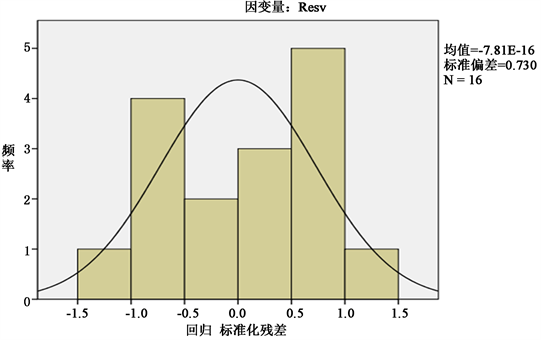Figure 1. Histogram of residual distribution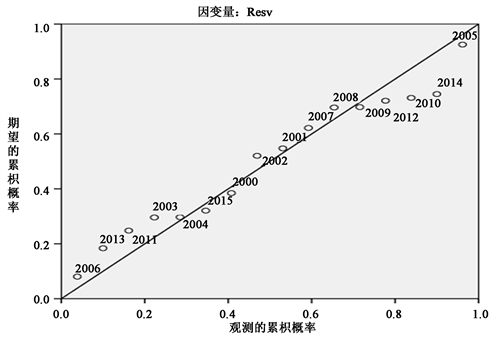Figure 2. Standard p-p diagram of regression standardization residuals

3.5. 实证结论

$\begin{array}{c}R{\text{esv}}_{\text{China}\left(\text{t}\right)}=-0.\text{826}+0.0\text{77}gd{p}_{t}+0.10ie{x}_{t}+0.0\text{30}fd{i}_{t}+0.0\text{00}kp{n}_{t}\\ \text{\hspace{0.17em}}\text{\hspace{0.17em}}+\text{0}\text{.806}stoc{k}_{\text{t}}+0.0\text{04}in{f}_{t}-0.01\text{1}ree{r}_{t}+0.\text{624}lagres{v}_{t}\end{array}$ (1)

3.6. 趋势预测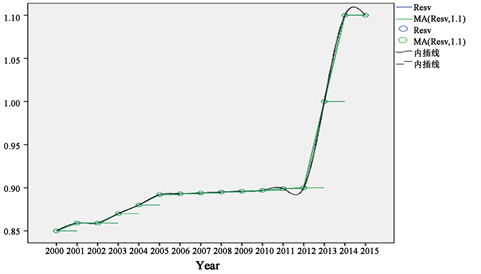Figure 3. Sequence diagram generated by the average moving center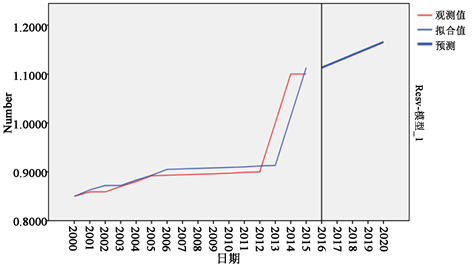Figure 4. Situation of measured values, fitting values and predicted values

4. 结语

An Empirical Analysis on the Determinants of the International Reserve Status of RMB[J]. 财富涌现与流转, 2018, 08(03): 28-38. https://doi.org/10.12677/ETW.2018.83004

1. 1. 李稻葵, 刘霖林. 人民币国际化: 计量研究及政策分析[J]. 金融研究, 2008(11): 1-16.

2. 2. Menzie, C. and Jeffrey, F. (2007) Will the Euro Eventually Surpass the Dollar as Leading International Reserve Currency? NBER Working Paper No .11510.

3. 3. 邓黎桥. 人民币国际化:影响因素、政策配合与监管[J]. 重庆大学学报(社会科学版), 2016, 22(1): 67-73.

4. 4. 王珊珊, 黄梅波, 陈燕鸿. 金融市场发展对一国储备货币地位的影响与人民币国际化——基于PSTR模型的实证分析[J]. 重庆大学学报(社会科学版), 2018(1): 22-33.

5. 5. 丁剑平, 楚国乐. 货币国际化的影响因子分析——基于面板平滑转换回归(PSTR)的研究[J]. 国际金融研究, 2014, 332(12): 35-46.

6. 6. 李建国. 人民币国际化制约因素及推进措施[D]: [博士学位论文]. 长春: 东北师范大学, 2014.

7. 7. 丁一兵, 付林. 金融市场发展影响货币国际地位的实证分析[J]. 吉林师范大学学报(人文社会科学版), 2014, 42(2): 101-106.

8. 8. 郝宇彪, 田春生. 人民币国际化的关键: 基于制约因素的分析[J]. 经济学家, 2011(11): 64-72.